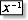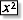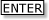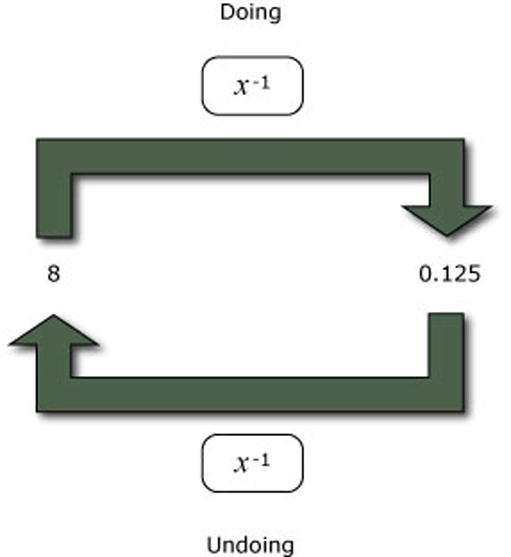Tapping into mathematics

Start this free course now. Just create an account and sign in. Enrol and complete the course for a free statement of participation or digital badge if available.

Free course

# 7.4 Reciprocals

There is a key on most scientific and graphics calculators which will give the reciprocal of a number. This is one over the number. So the reciprocal of 2 isor 0.5. The reciprocal of 4 isor 0.25. On many calculators this is labelled 1/x or, as on the TI-84, it is labelled x−1, which can be said ‘x to the power minus 1’ or ‘x to the minus 1’.

In general if you want the reciprocal of a number, you input the number and then press thekey (in the same way that you input the number and then pressto get the square of a number). If you press 8, you should get the reciprocal of 8, which is 0.125. If you press .123, you should get the reciprocal of 0.123, which is 8.130081301.

Now try inputting a number and pressing the reciprocal key twice. You should find that you get the input number. So what is the key which undoes the reciprocal? Here are some examples to help you

2gives .5. So the reciprocal of 2 is 0.5.

.5gives 2. So the reciprocal of 0.5 is 2.

8gives .125. So the reciprocal of 8 is 0.125.

.125gives 8. So the reciprocal of 0.125 is 8.

The reciprocal key undoes itself – the function x−1 is its own inverse.Figure 9 Doing and undoing reciprocals
MU120_4MCAL